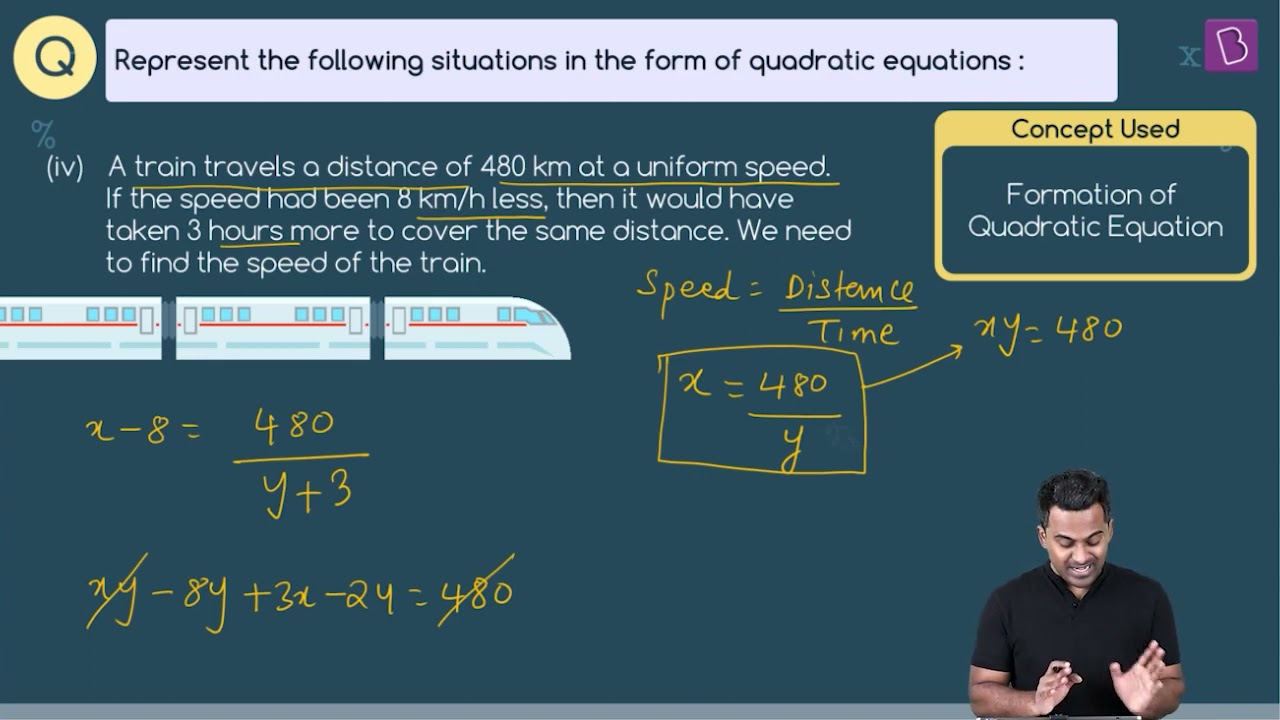# A train travels a distance of 480 km at a uniform speed. If the speed had been 8 km/h less, then it would have taken 3 hours more to cover the same distance. Find the speed of the train.Distance travelled by the train = 480 km

Let the speed of the train be x kmph

Time taken for the journey = 480/x

Given speed is decreased by 8 kmph

Hence the new speed of train = (x – 8) kmph

Time taken for the journey = 480/(x – 8)

480/(x – 8) – 480/x = 3

480(x – x + 8)/x (x-8) = 3

480/3 * 8 = x2 -8x

1280 = x2 – 8x

x2 – 8x – 1280 = 0

On solving we get x = 40

Thus the speed of train is 40 kmph(155)(92)